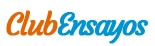# Registro De Pozos

Enviado por   •  12 de Septiembre de 2011  •  1.648 Palabras (7 Páginas)  •  705 Visitas

Página 1 de 7

Chapter 2

2.1 Structure of the Atom

The atom is the smallest particle 01 exist alone or in association with o of a nucleus and one or more orbits

The atom is the smallest particle of a chemical element that can exist alone or in association with other atoms. An atom consists of a nucleus and one or more orbital electrons. An electron, e ~, is a particle that is 9.1085 x 10 ~31 kg in mass and carries a nega¬tive charge of 1.60219xl0-19 C. Electrons occupy shells desig¬nated by the letters K, L, M, N, etc. Each shell can contain only a limited number of electrons. For example, the K shell, the first shell surrounding the atom, can contain only two electrons. The electron structure determines the chemical characteristics of the atom.

The nucleus is made of a combination of protons, p +, and neu¬trons, n. The proton is a particle with a mass that is 1,836 times the mass of an electron and carries a positive electric charge equal in magnitude to the negative charge of an electron. The number of protons in a nucleus is called the atomic number, Z. A neutral atom of atomic number Z will have 2 orbital electrons. The neu¬tron is an electrically neutral particle with a mass that is 1,838 times the mass of an electron. The sum of the number of protons and neutrons in the nucleus is the mass number, A.

An atom's mass is commonly given relative to the atom of oxy¬gen, which is arbitrarily assigned the value of 16.0 atomic mass units (amu). The atomic mass rounded to the nearest integer equals the mass number, A. The atomic mass is then usually assigned the same symbol, A. The sum of the atomic mass of all the atoms that make up a molecule defines its molecular mass, M. By definition, 1 amu = J/[6 (mass of an atom of oxygen) or 1 amu = {/32 (mass of a molecule of oxygen).

According to Avogadro's law, a gram-molecular weight (gmol) of any substance contains the same number of molecules. This num¬ber, NA, known as Avogadro's number, is 6.02252X1023 molecules/gmol. Thus,

1 / 1 gmol 1 amu = —

32 \6.02252xl023

A mole of a substance is the number of mass units of that sub¬stance equal to molecular mass. A gmol of oxygen is equivalent to 32 g, and

gmol

1/22 g

1 amu=— ,,

32 V gmol A 6.02252 XlO23

= 1.6604xl0"24 g = 1.6604xl0~27 kg.

Also, the number of atoms per unit volume, Na, and the number of electrons per unit volume, Ne, can be expressed by

Na=NA{pIA) (2.1)

andNe=NaZ=NA{ZIA)p, (2.2)

where p is the bulk density in g/cm3.

A nucleus is usually described by ZEA where E is the chemical symbol of the element, Z is the atomic number (number of pro¬tons), and ,4 is the mass number (number of protons and neutrons). This form is convenient for balancing nuclear equations used to describe nuclear reactions.

The nucleus is small with respect to the atom's external dimen¬sion. An atom can be visualized as a space of which very little is occupied by mass. Fig. 2.1 illustrates the structure of some simple atoms.

Each specific combination of neutrons and protons is called a nuclide. Nuclides with the same atomic number, Z, but different mass numbers, A (i.e., different numbers of neutrons), are called isotopes of the same element. For example, ^W, iH2,and tH3 are isotopes of the element hydrogen.

jH2 is called deuterium or heavy hydrogen and [H3 is called tritium. All three have an atomic number of one; i.e., they all con¬tain one proton. The atomic masses of the three isotopes are 1, 2, and 3, respectively. The proportions of jH1 and [H2 in nature are 99.9844% and 0.0156%, respectively. ]H3 exists in very minute proportions. It is continuously created in the upper atmosphere by cosmic rays.'

The number of isotopes of an element and their proportions are different for every element. For instance, uranium has two natural isotopes, 92U235 and 92U238, with relative abundances of 0.7% and 99.3%, respectively. Table 2.1 lists properties of common nuclides.

The bonding forces that "glue" the nucleons (i.e., protons and/or neutrons) together are provided by the "meson" particles. Meson particles are unstable complex structures that may be charged posi¬tively or negatively or may be neutral.

The bonding system of nucleons is characterized by a set of dis¬crete energy levels or states. Each nuclide has a unique set of ener¬gy states. A stable nucleus exists at the lowest energy level, known as the ground state. It can reach higher levels, known as excited states, by appropriate insertion of energy. A nuclide in an excited state is unstable and must release the excess energy so that it can

26

IHtUHY, MfcASUHfcMfcN I , AINU IN I fcHKHb IA IIUN Uh WtLL LUUb

,,, — — •*

s N

s *

/ X

t \

V \

J

V ' 4

^---^ Ho

HELIUM 2

_^ —• ——• m. ■«N

*"* N.

s N

/ ,'—-

I / • \

\ \

/ / __ i \

' / JsJ*S-v \ »

N *^-. - ' ;

--V

\ /

"- ^ Li

LITHIUM^

...

Descargar como (para miembros actualizados)  txt (11.3 Kb)
Leer 6 páginas más »
Disponible sólo en Clubensayos.com